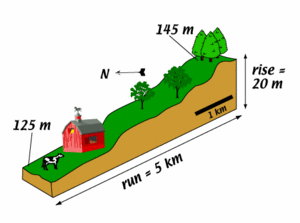# How do I calculate slope/gradient? "Rise over run" in the geosciencesMany of us know that the slope of a line is calculated by "rise over run". However, the application of slope calculation can seem a little more complicated. In the geosciences, you may be asked to calculate the slope of a hill or to determine rate by calculating the slope of a line on a graph. This page is designed to help you learn these skills so that you can use them in your geoscience courses.

## Why should I calculate slope or gradient?

In the geosciences slope can play an important role in a number of problems. The slope of a hill can help to determine the amount of erosion likely during a rainstorm. The gradient of the water table can help us to understand whether (and how much) contamination might affect a local well or water source.

## How do I calculate slope (or gradient) in the geosciences?

Gradient in the case of hillslope and water table is just like calculating the slope of a line on a graph - "rise" over "run". But how do you do that using a contour (or topographic) map?

1. First get comfortable with the features of the topographic map of interest. Make sure you know a few things:
• What is the contour interval (sometimes abbreviated CI)?
• What is the scale of the map?
• What is the feature for which you want to know the slope?
2. First, you need to know "rise" for the feature. "Rise" is the difference in elevation from the top to bottom (see the image above). So determine the elevation of the top of the hill (or slope, or water table).
3. Next you need to know "run" for the feature. "Run" is the horizontal distance from the highest elevation to the lowest. So, get out your ruler and measure that distance. If you know the scale, you can calculate the distance. Most of the time distance on maps is given in km or mi.
4. Now comes the rise over run part. There are two ways that you may be asked to make calculations relating to slope. Make sure you know what the question is asking you and follow the steps associated with the appropriate process: